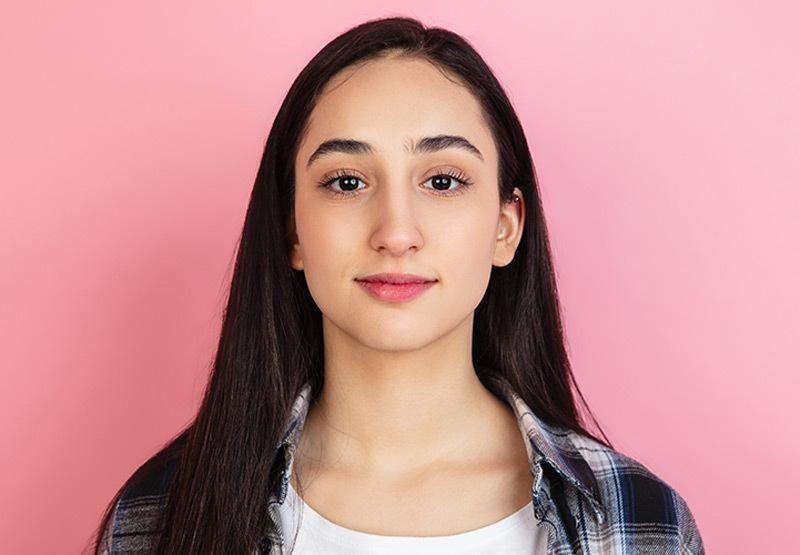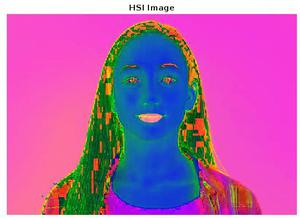GeeksforGeeks App
Open AppBrowser
Continue

# How to Converting RGB Image to HSI Image in MATLAB?

Converting the color space of an image is one of the most commonly performed operations in Image Processing. It is used so much as a lot of transformation/filters are performed on a specific color mode of an image. i.e. Thresholding is performed in Grayscale, Color slicing in HSV etc. A color space that has received a lot of attention overtime is HSL (Hue Saturation Intensity). This is because of the information that is obtained about the image and its contents just by the analysis of the channels. Therefore, it might be required to convert the image to HSI color space to perform some operation. Unfortunately, MATLAB does not have any built-in function for performing conversions to HSI color mode. So in this article, you will learn how to convert an RGB image to HSI image in MATLAB.

Example 1:

## Matlab

 `% MATLAB program for RGB to HSI image conversion.``    ``img = imread(``'test.jpg'``);` `% Represent the RGB image in [0 1] range``    ``I = double(img) / 255;``    ``R = I(:,:,1);``    ``G = I(:,:,2);``    ``B =    I(:,:,3);` `% Converting the image to HSV to``% obtain the Hue and Saturation Channels``    ``HSV = rgb2hsv(img);``    ``H = HSV(:,:,1);``    ``S = HSV(:,:,2);` `% Intensity``    ``I = sum(I, 3)./3;` `% Creating the HSL Image``  ``HSI = zeros(size(img));``  ``HSI(:,:,1) = H;``  ``HSI(:,:,2) = S;``  ``HSI(:,:,3) = I;``  ``figure,imshow(HSI); title(``'HSI Image'``);`

Output:

We would be using the following image for demonstration:Explanation:

Firstly an RGB image named test.jpg is imported. Then all the values of each channel are divided by 255, giving us binary values which are stored in the variable I. Each channel of the RGB image is then obtained and stored in different variables. Then the image is converted to HSV color space using rgb2hsv function. This will allow the use of Hue and Saturation channels that are obtained from this conversation (would ease the process of getting the Hue and Saturation Channels). Then Hue and Saturation channels are stored in separate variables as well. Then the intensity of the image is obtained using the formula:Then a zero matrix is created of the same size as the original image, and then populated with the Hue, Saturation and Intensity Channels. In the end, the HSI image is displayed.

My Personal Notes arrow_drop_up